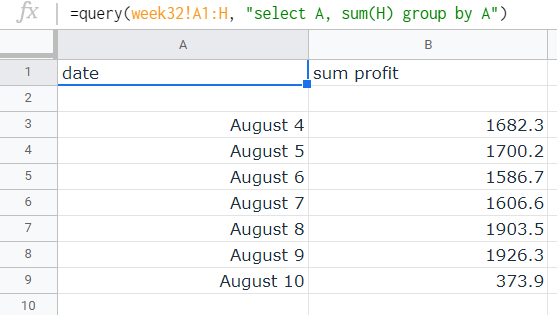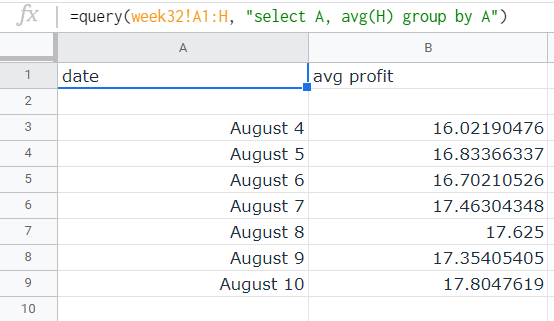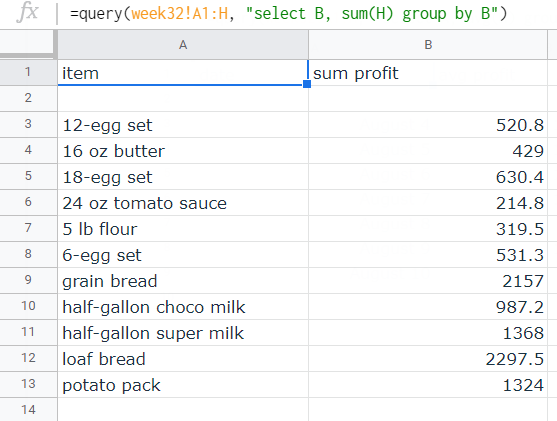October 15, 2020

# How to Use the 'Group by' SQL Clause in Google SheetsThe group by clause is a powerful clause in the QUERY function that actually lets you further summarize large amounts of data in Google Sheets. In this tutorial, we will explore how to get the aggregate values (click here for a review of aggregate functions that can be used in queries) and calculate them by group through the SQL-like Query Language in Google Sheets.

## Group by date

The most common way of grouping the rows of entries would be by grouping them by the date. To do so, add the aggregate function applied to the row then followed by group by clause followed by the column containing the date. As an example, we have the following sheet that includes the date of transaction, the item bought, the price per item, the amount of items bought, the resulting total sales, the cost per item, the total cost, and the profit.

We can calculate the total profit per day. To do so, we can use the following query:

select A, sum(H) group by A

With this, we'll have to place it in Google Sheets' QUERY function with the format:

=query([range],"[SQL query]'")

And the result will look like this:Results of the query. The first column contains the specific date; the second column contains the “sum profit” per date listed.

Pretty neat, right? All five aggregate functions will work in the given format. For example, you can also calculate the average profit per day using the following query:

select A, avg(H) group by A

With this, we'll have to place it in Google Sheets' QUERY function with the format:

=query([range],"[SQL query]'")

And the result will look like this:Results of the query. The first column contains the specific date; the second column contains the “avg profit” per date listed.

Unfortunately, the group by clause does not allow you to further group the data by specific month or year unless the data originally contains the specific rows for the month or year. In order to complete that task, you will first have to do this in the original sheet. You can check the latter part of this tutorial to know how to do so in the original sheet.

## Group by item

The group by clause also works like charm if you want to group the orders by item. For example, the orders that we have are orders of one of the eleven products. We can find out which is giving us the biggest profit by calling the column containing the product and then using the same query we used above:

select B, sum(H) group by B

With this, we'll have to place it in Google Sheets' QUERY function with the format:

=query([range],"[SQL query]'")

The results look like this:Results of the query. The first column contains the specific item; the second column contains the “sum profit” per listed item.

## A bit of a hassle?

While this lets us analyze large chunks of data much easier, it will take us a lot of time to do so. Maybe there is a better way so that all the analysis is automated and we will just have to make important decisions for our business…

If that’s what you fancy, consider trying Lido. With a few clicks, you can now access all the relevant metrics without going through the hassle of accessing the SQL databases of your eCommerce platforms and then coding the formulas to process them. Let our platform do it all for you!Suscribe to get more data and analytics tips!